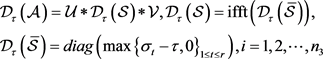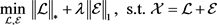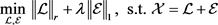﻿ 基于截断核范数的张量去噪

# 基于截断核范数的张量去噪Tensor Denoising Based on Truncated Nuclear Norm

Abstract: Based on the problem of tensor nuclear norm, a new robust principal component analysis model is established by substituting truncated nuclear norm for nuclear norm, and the convex optimization problem is solved by augmented Lagrange multiplier method. In the experiment of image denoising, the robust principal component analysis model with truncated unclear norm has good denosing effect.

1. 引言

2. 预备知识

${‖\mathcal{A}‖}_{\ast }:=\frac{1}{{n}_{3}}{\sum }_{i=1}^{{n}_{3}}{‖{\stackrel{¯}{A}}^{\left(i\right)}‖}_{\ast }$ (1)

${‖\mathcal{X}‖}_{r}={‖\mathcal{X}‖}_{\ast }-\underset{\begin{array}{l}\mathcal{A}\ast {\mathcal{A}}^{\text{T}}=\mathcal{I}\\ \mathcal{B}\ast {\mathcal{B}}^{\text{T}}=\mathcal{I}\end{array}}{\mathrm{max}}tr\left(\mathcal{A}\ast \mathcal{X}\ast \mathcal{B}\right)$ (2)

$\underset{i=1,\cdots ,{n}_{1}}{\mathrm{max}}{‖{\mathcal{U}}^{\ast }\ast \stackrel{\circ }{{\mathfrak{e}}_{i}}‖}_{F}\le \sqrt{\frac{\mu r}{{n}_{1}{n}_{3}}}$ (3)

$\underset{j=1,\cdots ,{n}_{2}}{\mathrm{max}}{‖{\mathcal{V}}^{\ast }\ast \stackrel{\circ }{{\mathfrak{e}}_{j}}‖}_{F}\le \sqrt{\frac{\mu r}{{n}_{2}{n}_{3}}}$ (4)

${‖\mathcal{U}\ast {\mathcal{V}}^{\ast }‖}_{\infty }\le \sqrt{\frac{\mu r}{{n}_{1}{n}_{2}{n}_{2}^{3}}}$ (5)

$\mathcal{A}=\mathcal{U}\ast \mathcal{S}\ast {\mathcal{V}}^{\ast }$ (6)

$\mathcal{U}\in {ℝ}^{{n}_{1}×{n}_{1}×{n}_{3}},\mathcal{V}\in {ℝ}^{{n}_{2}×{n}_{2}×{n}_{3}}$ 是正交的， $\mathcal{S}\in {ℝ}^{{n}_{1}×{n}_{2}×{n}_{3}}$ 是f-对角张量。(7)

3. 截断核范数的鲁棒主成分分析

3.1. 模型的建立(8)(9)

3.2. 模型的求解

$\underset{X}{\mathrm{min}}\text{\hspace{0.17em}}\text{ }{‖X‖}_{\ast }-Tr\left({\mathcal{A}}_{l}\mathcal{W}{\mathcal{B}}_{l}^{\text{T}}\right)+\lambda {‖\mathcal{E}‖}_{1}\text{\hspace{0.17em}}\text{\hspace{0.17em}}\text{s}\text{.t}\text{.}\text{\hspace{0.17em}}\text{ }\mathcal{L}=\mathcal{W},\text{\hspace{0.17em}}\mathcal{X}=\mathcal{L}+\mathcal{E}$ (10)

$\begin{array}{c}L\left(\mathcal{L},\mathcal{E},\mathcal{W},{\mathcal{Y}}_{1},{\mathcal{Y}}_{2}\right)={‖\mathcal{L}‖}_{\ast }-Tr\left({\mathcal{A}}_{l}\mathcal{W}{\mathcal{B}}_{l}^{\text{T}}\right)+\lambda {‖\mathcal{E}‖}_{1}+〈{\mathcal{Y}}_{1},\mathcal{L}+\mathcal{E}-\mathcal{X}〉\\ \text{\hspace{0.17em}}\text{\hspace{0.17em}}+〈{\mathcal{Y}}_{2},\mathcal{L}-\mathcal{W}〉+\frac{\mu }{2}\left({‖\mathcal{L}+\mathcal{E}-\mathcal{X}‖}_{F}^{2}+{‖\mathcal{L}-\mathcal{W}‖}_{F}^{2}\right)\end{array}$ (11)

$\begin{array}{c}{\mathcal{L}}_{k+1}=\mathrm{arg}\underset{\mathcal{L}}{\mathrm{min}}\text{\hspace{0.17em}}{‖\mathcal{L}‖}_{\ast }+〈{\mathcal{Y}}_{1,k},\mathcal{L}+{\mathcal{E}}_{k}-\mathcal{X}〉+〈{\mathcal{Y}}_{2,k},\mathcal{L}-{\mathcal{W}}_{k}〉+\frac{\mu }{2}\left({‖\mathcal{L}+{\mathcal{E}}_{k}-\mathcal{X}‖}_{F}^{2}+{‖\mathcal{L}-{\mathcal{W}}_{k}‖}_{F}^{2}\right)\\ =\mathrm{arg}\underset{\mathcal{L}}{\mathrm{min}}\text{\hspace{0.17em}}{‖\mathcal{L}‖}_{\ast }+\mu {‖\mathcal{L}+\frac{1}{2}\left({\mathcal{E}}_{k}-\mathcal{X}-{\mathcal{W}}_{k}+\frac{1}{\mu }\left({\mathcal{Y}}_{1k}+{\mathcal{Y}}_{2k}\right)\right)‖}_{F}^{2}\end{array}$ (12)(13)

$\begin{array}{c}{\mathcal{E}}_{k+1}=\mathrm{arg}\underset{\mathcal{E}}{\mathrm{min}}\lambda {‖\mathcal{E}‖}_{1}+〈{\mathcal{Y}}_{1k},{\mathcal{L}}_{k+1}+\mathcal{E}-\mathcal{X}〉+\frac{\mu }{2}{‖{\mathcal{L}}_{k+1}+\mathcal{E}-\mathcal{X}‖}_{F}^{2}\\ =\mathrm{arg}\underset{\mathcal{E}}{\mathrm{min}}\lambda {‖\mathcal{E}‖}_{1}+\frac{\mu }{2}{‖\mathcal{E}+\left({\mathcal{L}}_{k+1}-\mathcal{X}+\frac{1}{\mu }{\mathcal{Y}}_{1,k}\right)‖}_{F}^{2}\end{array}$ (14)

${\mathcal{E}}_{k+1}={\mathcal{S}}_{\frac{\lambda }{{\mu }_{k}}}\left(\mathcal{X}-{\mathcal{L}}_{k+1}-\frac{1}{\mu }{\mathcal{Y}}_{1,k}\right)$ (15)

${\mathcal{W}}_{k+1}=\mathrm{arg}\underset{\mathcal{W}}{\mathrm{min}}\text{\hspace{0.17em}}\text{ }-Tr\left({\mathcal{A}}_{l}\mathcal{W}{\mathcal{B}}_{l}^{\text{T}}\right)+〈{\mathcal{Y}}_{2k},{\mathcal{L}}_{k+1}-\mathcal{W}〉+\frac{\mu }{2}{‖{\mathcal{L}}_{k+1}-\mathcal{W}‖}_{F}^{2}$ (16)

(16)式为关于 $\mathcal{W}$ 的二次项，令其导数为0，可得到闭式解。去掉无关项得：

${\mathcal{W}}_{k+1}=\mathrm{arg}\underset{\mathcal{W}}{\mathrm{min}}\text{\hspace{0.17em}}\text{ }-Tr\left({\mathcal{A}}_{l}\mathcal{W}{\mathcal{B}}_{l}^{\text{T}}\right)+\frac{\mu }{2}{‖\mathcal{W}‖}_{F}^{2}+〈-{\mathcal{Y}}_{2k},\mathcal{W}〉+\mu 〈-{\mathcal{L}}_{k+1},\mathcal{W}〉$ (17)

$-{\mathcal{A}}_{l}{\mathcal{B}}_{l}^{\text{T}}+\mu \mathcal{W}-{\mathcal{Y}}_{2k}-\mu {\mathcal{L}}_{k+1}=0$ (18)

$\mathcal{W}={\mathcal{L}}_{k+1}+\frac{1}{\mu }\left({\mathcal{A}}_{l}{\mathcal{B}}_{l}^{\text{T}}+{\mathcal{Y}}_{2k}\right)$ (19)

$\begin{array}{l}{\mathcal{Y}}_{1k+1}={\mathcal{Y}}_{1k}+\mu \left({\mathcal{L}}_{k+1}+{\mathcal{E}}_{k+1}-\mathcal{X}\right)\\ {\mathcal{Y}}_{2k+1}={\mathcal{Y}}_{2k}+\mu \left({\mathcal{L}}_{k+1}-{\mathcal{W}}_{k+1}\right)\end{array}$ (20)

4. 实验原始图片噪声图片TRPCATNNRSNNTNNRPCA

Figure 1. Comparison of denoising effects of different methodsTable 1. Data denoised by each algorithm

5. 结论

 Jolliffe, I. (2002) Principal Component Analysis. Wiley Online Library, New York.

 Bouwmans, T. and Zahzah, E.H. (2014) Robust PCA via Principal Component Pursuit: A Review for a Comparative Evaluation in Video Surveillance. Computer Vision and Image Understanding, 122, 22-34.

 Candès, E.J., Li, X., Ma, Y. and Wright, J. (2011) Robust Principal Component Analysis? Journal of the ACM (JACM), 58, 11.
https://doi.org/10.1145/1970392.1970395

 Xie, Y., Gu, S., Liu, Y., Zuo, W., Zhang, W. and Zhang, L. (2016) Weighted Schatten $p$-Norm Minimization for Image Denoising and Background Subtraction. IEEE Transactions on Image Processing, 25, 4842-4857.
https://doi.org/10.1109/tip.2016.2599290

 Hu, Y., Zhang, D., Ye, J., Li, X. and He, X. (2012) Fast and Accurate Matrix Completion via Truncated Nuclear Norm Regularization. IEEE Transactions on Pattern Analysis and Machine Intelligence, 35, 2117-2130.
https://doi.org/10.1109/tpami.2012.271

 Kilmer, M.E. and Martin, C.D. (2011) Factorization Strategies for Third-Order Tensors. Linear Algebra and Its Applications, 435, 641-658.
https://doi.org/10.1016/j.laa.2010.09.020

 Lu, C., Feng, J., Chen, Y., Liu, W., Lin, Z. and Yan, S. (2016) Tensor Robust Principal Component Analysis: Exact Recovery of Corrupted Low-Rank Tensors via Convex Optimization. 2016 Proceedings of the IEEE Conference on Computer Vision and Pattern Recognition, Las Vegas, NV, 27-30 June 2016, 5249-5257.
https://doi.org/10.1109/cvpr.2016.567

 Xue, S., Qiu, W., Liu, F. and Jin, X. (2017) Low-Rank Tensor Completion by Truncated Nuclear Norm Regularization. arXiv preprint arXiv:1712.00704
https://doi.org/10.1109/icpr.2018.8546008

Top